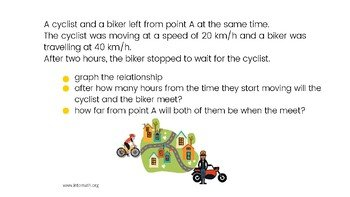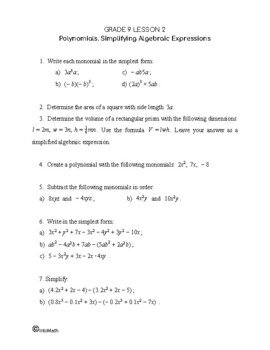CA\$2.00

In this lesson you will learn about linear relations and what they mean. One of the most common examples of a linear relation is the relationship between distance and time when a distance changes over time at a constant rate.

A linear relation is represented by a straight-line – a relationship between a variable and a constant. Linear relations can be expressed in 4 different ways: a graph, a table of values, a word description and an equation.